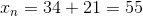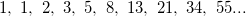# High School Math : Understanding Sequences

## Example Questions

### Example Question #1 : Summations And Sequences

Consider the Fibonacci sequence written below. What are the next three terms?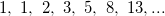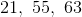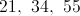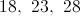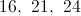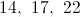Explanation:

Each number in the Fibonacci sequence can be calculated by the sum of the previous two numbers.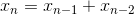The next term in the sequence will be the sum of the two terms preceding it: 8 and 13.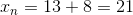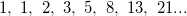The next term will be the sum of 13 and 21.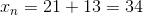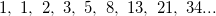The next term will be the sum of 21 and 34.